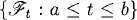# THE ITÔ INTEGRAL

The Itô integral defines an integral of a stochastic process with respect to a standard Brownian motion. The Itô integral is more general than the Wiener integral introduced in the previous chapter. In this chapter, we shall introduce the concept of Itô integrals.

# 32.1 Basic Concepts and Facts

Definition 32.1 (Stochastic Process Space L2ad([a, b] × Ω)). Letbe a filtration under consideration. The space L2ad([a, b] × Ω) is defined to be the space of all stochastic processes f(t, ω), t[a, b], ωΩ, satisfying the following conditions:

(a) f(t) is adapted to thet.
(b)ba E(|f(t)|2)dt < ∞.

Definition 32.2 (Itô Integral of Step Stochastic Processes). Let {t : atb} be a filtration. Let {Bt : atb} be a Brownian motion satisfying the following conditions:

(a) For each t, Bt is t-measurable.
(b) For any st, the random variable BtBs is independent ...

Get Measure, Probability, and Mathematical Finance: A Problem-Oriented Approach now with the O’Reilly learning platform.

O’Reilly members experience live online training, plus books, videos, and digital content from nearly 200 publishers.